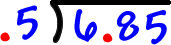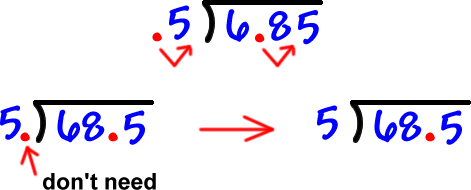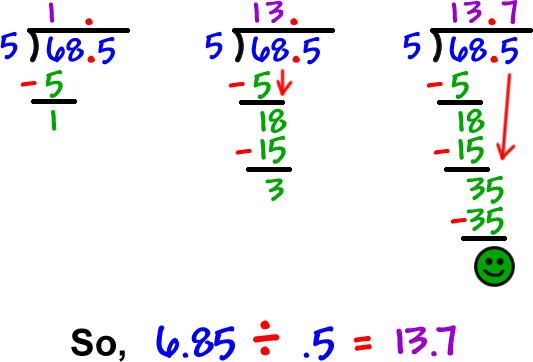So, how do we do a decimal division problem like 6.85 divided by .5?

Well, we already know how to divide a decimal by a whole number -- and it was pretty easy....

So, let's just turn these problems into the easy kind!

How do we do that?  It looks like it's just a trick...  But, I'll show you why it works!

Let's divide 6.85 by .5

Set it up:Move the decimals to the right on both until you are dividing BY a whole number:Now, go for it!>> Remember, you can check this by multiplying

13.7 x .5